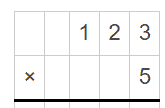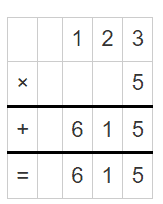# 3 digit by 1 digit Multiplication Worksheets

• ###### 3 digit by 1 digit Multiplication #15

These worksheets were designed to help you practice and learn how to multiply numbers like 123 (a three-digit number) by 7 (a one-digit number). Mastering this will elevate your math skills, making you quicker and more accurate in solving multiplication problems, and not to mention, it will make math feel more like an exciting treasure hunt rather than a daunting task.

Now, let’s explore the different types of exercises you’ll find on these worksheets. Each one has been designed like an intriguing puzzle or a captivating game, helping you unravel the mysteries of multiplication one step at a time.

Vertical Multiplication Problems – These are quite straightforward. You’ll see a three-digit number atop a one-digit number with a multiplication symbol (x) on the side. Your job is to multiply these numbers. This exercise makes you familiar with the process of 3-digit by 1-digit multiplication, gradually making you more adept at it.

Fill in the Blanks – In this type of problem, a part of the multiplication equation is missing, and you need to find it. For example, 123 x _ = 861. These puzzles challenge you to think in reverse, thereby deepening your understanding of multiplication.

Mixed Review Problems – These are a blend of different types of problems, like word problems, fill in the blanks, and straightforward multiplication problems. These problems test your overall understanding and are great for reinforcing what you’ve learned.

So, how do these worksheets and exercises improve your math skills? Let’s delve into that.

The heart of multiplication is repeated addition. So, by understanding how to multiply a three-digit number by a one-digit number, you’re strengthening your understanding of this core mathematical concept. As you practice, you’ll become quicker and more accurate with multiplication, which will help when you tackle more complex math problems. In fact, the ability to multiply quickly and accurately will make it easier for you to understand more advanced math topics in the future, like algebra, which relies heavily on multiplication.

The fill in the blank problems prompt you to think more deeply about multiplication and how it works. This deeper understanding can make you more confident in your math abilities and make math more fun.

And mixed review problems are great because they help cement everything you’ve learned. They provide a good recap and challenge you to remember what you’ve learned in previous exercises.

These worksheets offer a variety of engaging exercises that help you practice and understand multiplication in fun and different ways. Through this practice, you’re not only improving your speed and accuracy but also understanding how multiplication is used in real-life situations and developing valuable problem-solving skills.

### How to Solve These Types of Problems

Multiplying a three-digit number by a single digit number may seem complex, but once you get a grasp on the steps, it becomes a simple process. Let’s go through it using an example – 123 x 5.

Step 1) Setting up the Problem – Write the numbers vertically, with the larger number (123) on top and the smaller number (5) underneath. Make sure to align the digits by place value (ones, tens, hundreds).Step 2) Multiply the Ones – Start with the ones place. Multiply the digit in the ones place of the bottom number (5) by the digit in the ones place of the top number (3). So, 5 x 3 = 15. Write ‘5’ below the line and carry over the ‘1’ to the next place value, the tens place.

Step 3) Multiply the Tens – Next, multiply the bottom number (5) by the digit in the tens place of the top number (2). So, 5 x 2 = 10. Then add the ‘1’ you carried over. So, 10 + 1 = 11. Write down ‘1’ and carry over the other ‘1’ to the hundreds place.

Step 4) Multiply the Hundreds – Now, multiply the bottom number (5) by the digit in the hundreds place of the top number (1). So, 5 x 1 = 5. Don’t forget to add the ‘1’ you carried over. So, 5 + 1 = 6. Write this down.So, 123 multiplied by 5 equals 615. Remember, it’s all about multiplying each digit and carrying over any value greater than 9 to the next place value. With a little bit of practice, you’ll soon be able to multiply three-digit numbers by a single-digit number quickly and accurately!